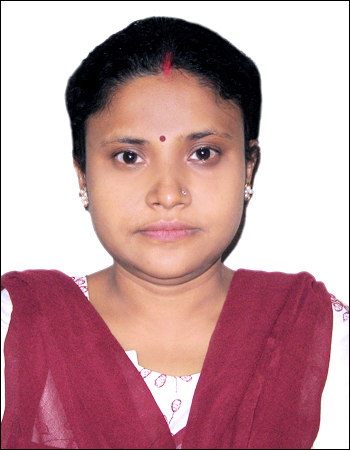# Partial Differential Equations

By Prof. Alaka Das   |   Jadavpur University
Learners enrolled: 1858

Partial differential equations (PDE) is an important branch of Science. It has many applications in various physical and engineering problems. Thus the proposed course  is helpful to the learners from Mathematics, Physics and Engineering background.

In this course mainly we have attempted to give some ideas about first order and second order linear PDE. Nonlinear PDE is discussed in the last Chapter shortly. The method of solving first-order and second order equations are illustrated taking many examples. There are also problems for self- assessment with answer. The entire course is divided into ten Chapters. Each Chapter is broken into few modules. The video and text of the modules are available on enrolment to this course. The last date for enrolment is 31st  August, 2020. The enrolment to this course is free of cost.

If the leaner find any difficulty to understand some problem, that can be discussed in the discussion forum in which course coordinator is also a member.

After completion of this course one may apply for a certificate.

Summary
 Course Status : Ongoing Course Type : Core Duration : 15 weeks Start Date : 06 Jul 2020 End Date : 14 Dec 2020 Exam Date : Enrollment Ends : 14 Oct 2020 Category : Mathematics Level : Postgraduate

### Course layout

Chapter 1- Basic Concepts of Partial Differential Equations

Chapter 2- First Order Partial Differential Equations

Chapter 3- Second Order Partial Differential Equations

Chapter 4- Elliptic Differential Equations

Chapter 5- Parabolic Differential Equations

Chapter 6- Hyperbolic Differential Equations

Chapter 7- Integral Transform Methods of Solving Partial Differential Equations

Chapter 8- Green’s Function of solving Partial Differential Equations

Chapter 9- Eigenfunction Approach

Chapter 10- Nonlinear Partial Differential Equations

Week 1: Chapter 1

Week 2: Chapter 1 and 2

Week 3: Chapter 2

Week 4: Chapter 3

Week 5: Chapter 4

Week 6: Chapter 5

Week 7: Chapter 6

Week 8: Revision of Chapter 1-6

Week 9: Chapter 7

Week 10: Chapter 7

Week 11: Chapter 8

Week 12:Chapter 9

Week 13: Chapter 10

Week 14: Chapter 10

Week 15: Revision of Chapter 7-10 and Assessment from the entire syllabus

### Books and references

  Carrier, G. F. and Pearson, C.F.: Partial Differential Equations -Theory and Technique, Academic Press, New York (1976).  Chester, C. R. : Tecniques in Partial Differential Equations, Macgraw-Hill,NewYork (1971).  Duchaleau, P. and Zachmann, D.W.: Partial Differential Equations, (Schaum’s Outline Series in Mathematics), McGraw -Hill, NewYork (1968).  Greenberg, M. : Applications of Green’s Functions in Science and Engineering, Prentice -Hall, Eaglewood Cliffs, New Jersey (1971).  John. F. : Partial Differential Equations, Springer -Verlag, NewYork (1971).  Machie, A. G. : Boundary Value Problems, Oliver & Boyd, London (1965).  Mynt -U, T. : Partial Differential Equations of Mathematical Physics, American Elesevier Publishing Co, Inc. (1973).  Mynt -U, T and Debnath, L. : Linear Partial Differential Equations for Scientists and Engineers, Birkhäuser, Boston (2007).  Rao, K. Sankara : Introduction to Partial Differential Equations, Prentice-Hall of India Private Limited, New Delhi (1997).  Sanyal. D.C. : Partial Differential Equations, (Study Material), Netaji Subhas Open University, Kolkata.  Sharma, J. N. and Sing, K. : Partial Differential Equations for Engineers and Scientists, Narosa Publishing House, New Delhi (2001).  Sneddon, I. N. : Elements of Partial Differential Equations, McGraw Hill Book Co, Singapur (1986).  Stephenson, G. : An Introduction to Partial Differential Equations for Science Students, ELBS series & Longman, London (1977).  Tychonov, A. N. and Samarki, A. A. : Partial Differential Equations of Mathematical Physics, Vol -I, Holden -Day, London (1964).  Weinberger, H. F. : A first Course in Partial Differential Equations, Blaisdell Publishing Co., New York (1965).

### Instructor bio### Prof. Alaka Das

Educational Qualification: M.Sc(Applied Mathematics), Ph.D (2006)

Teaching Experience: 19 years in UG and PG level

Research Interest: Hydrodynamic and hydromagnetic convection, Dynamical systems, nonlinear dynamics, Differential equations, Numerical Analysis

Research Experience: 22 years

### Course certificate

At the end of this course an examination will be conducted  by CEC, UGC. The date of examination is 15th November, 2020.  After successful completion of examination, a certificate will be issued by the host University after payment of registration fees. All information will be notified in the SWAYAM platform.